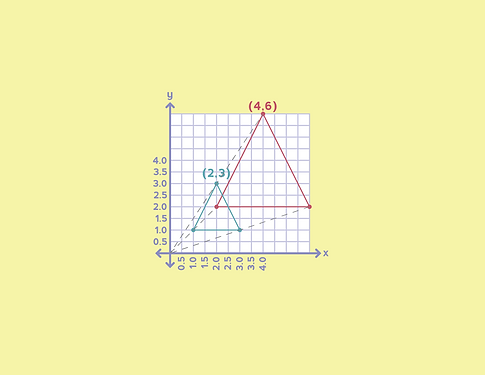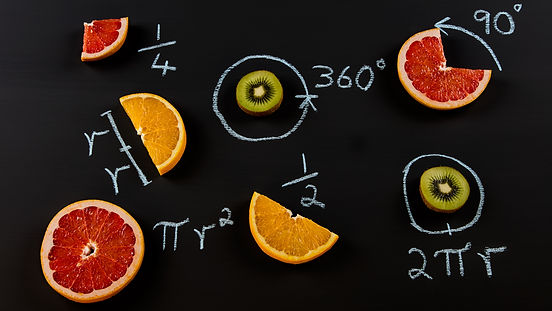top of page## GEOMETRY

Growing IQ first explores geometry through basic shape recognition and non-standard measurement. As knowledge and familiarity increase, the students are introduced to more complex concepts such as angles, lines, rays, and plane geometry.

Geometry is everywhere in architecture, mechanical engineering, design, art, and many other aspects of our 3D world.## 7 Categories of Geometry## CURRENCY

Money is an important part of the world.  A firm grasp of coin recognition, counting coins, making change, and writing monetary values is needed to navigate through life.  Solving currency problems requires skills in decimal arithmetic, skip counting, and an understanding of fractional numbers.## Learning how to tell time encapsulates a variety of skills that are essential in other areas of mathematics like calculating elapsed time; skip counting; differentiating between hours/minutes/seconds; and matching numbers and hours.## MEASUREMENT

Measuring distance, angles, area, and volume are all necessary skills for solving real-world problems.  Measurement provides opportunities to strengthen both number and geometric understandings; while allowing for meaningful comparisons of objects by attribute.## Coordinates are a set of values that express the exact position of an object in a coordinate plane. Coordinate understandings are essential in geometry, algebra, functions, and calculus.## CONGRUENCE

Congruence is the study of comparing two figures to show geometric agreement and correspondence.  Determining congruence draws conclusions about shapes and is the basis for many geometric theorems.## Studying symmetry enables recognition of the inherent patterns of objects; focusing on the characteristics and parts of objects, strengthening spatial reasoning skills, and is an integral component that connects math to the real-world.## SHAPES

Exploring shapes builds skills in recognition, classification, problem-solving, describing spatial relationships, composition, decomposition, and visualization.  Building these skills at an early age, sets a foundation for the more advanced geometry concepts taught in later years.

bottom of page